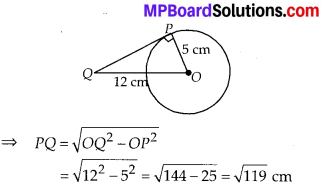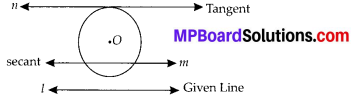In this article, we will share MP Board Class 10th Maths Book Solutions Chapter 10 Circles Ex 10.1 Pdf, These solutions are solved subject experts from the latest edition books.

## MP Board Class 10th Maths Solutions Chapter 10 Circles Ex 10.1

Question 1.
How many tangents can a circle have?
Solution:
A circle can have an infinite number of tangents.

Question 2.
Fill in the blanks :
(i) A tangent to a circle intersects it in _________ point(s).
(ii) A line intersecting a circle in two points is called a ______.
(iii) A circle can have ______ parallel tangents at the most.
(iv) The common point of a tangent to a circle and the circle is called _______.
Solution:
(i) Exactly one
(ii) Secant
(iii) Two
(iv) Point of contactQuestion 3.
A tangent PQ at a point P of a circle of radius 5 cm meets a line through the centre O at a point Q so that OQ = 12 cm. Length PQ is
(A) 12 cm
(B) 13 cm
(C) 8.5 cm
(D) $$\sqrt{119}$$ cm
Solution:
In right ∆QPO,
OQ2 = OP2 + PQ2Question 4.
Draw a circle and two lines parallel to a given line such that one is a tangent and the other, a secant to the circle.
Solution:
We have the required figure, as shownHere, l is the given line and a circle with centre O is drawn.
Line n is drawn which is parallel to l and tangent to the circle. Also, m is drawn parallel to line 1 and is a secant to the circle.